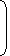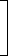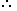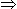# Tricks to Solve Problems on Percentage1. Concept of Percentage:By a certain percent, we mean that many hundredths.Thus, x percent means x hundredths, written as x%.
 To express x% as a fraction: We have, x% = x . 100
 Thus, 20% = 20 = 1 . 100 5
 To express a as a percent: We have, a =a x 100%. b b b
 Thus, 1 =1 x 100% = 25%. 4 4
2. Percentage Increase/Decrease:If the price of a commodity increases by R%, then the reduction in consumption so as not to increase the expenditure is:R x 100% (100 + R)

If the price of a commodity decreases by R%, then the increase in consumption so as not to decrease the expenditure is:R x 100% (100 – R)
3. Results on Population:Let the population of a town be P now and suppose it increases at the rate of R% per annum, then:
 1. Population after n years = P1 + Rn 100
2. Population n years ago = P1 + Rn 100
4. Results on Depreciation:Let the present value of a machine be P. Suppose it depreciates at the rate of R% per annum. Then:
 1. Value of the machine after n years = P1 – Rn 100
2. Value of the machine n years ago = P1 – Rn 100
 3. If A is R% more than B, then B is less than A byR x 100%. (100 + R)
 4. If A is R% less than B, then B is more than A byR x 100%. (100 – R)
A batsman scored 110 runs which included 3 boundaries and 8 sixes. What percent of his total score did he make by running between the wickets?
A. 45%
B.
 45 5 % 11
C.
 54 6 % 11
D. 55%

Explanation:

Number of runs made by running = 110 – (3 x 4 + 8 x 6)

= 110 – (60)

= 50.Required percentage =50 x 100% = 45 5 % 110 11
Two students appeared at an examination. One of them secured 9 marks more than the other and his marks was 56% of the sum of their marks. The marks obtained by them are:
 A. 39, 30 B. 41, 32 C. 42, 33 D. 43, 34

Explanation:

Let their marks be (x + 9) and x.

 Then, x + 9 = 56 (x + 9 + x) 10025(x + 9) = 14(2x + 9)3x = 99x = 33

So, their marks are 42 and 33.

#### A fruit seller had some apples. He sells 40% apples and still has 420 apples. Originally, he had:

Correct! Wrong!

#### What percentage of numbers from 1 to 70 have 1 or 9 in the unit's digit?

Correct! Wrong!

#### If A = x% of y and B = y% of x, then which of the following is true?

Correct! Wrong!

#### If 20% of a = b, then b% of 20 is the same as:

Correct! Wrong!

#### Two numbers A and B are such that the sum of 5% of A and 4% of B is two-third of the sum of 6% of A and 8% of B. Find the ratio of A : B.

Correct! Wrong!

QUIZ ON PERCENTAGE RELATED PROBLEMS
GREAT JOB!!!!